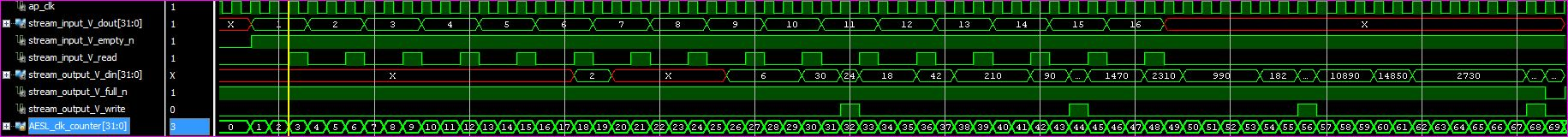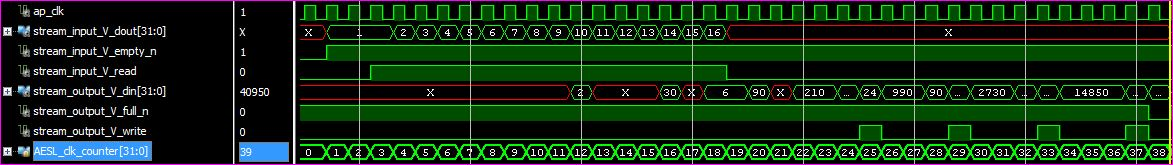# AR# 57710

## Description

An Initiation interval of 1 (ie II=1) for a top level loop with the "PIPELINE" directive was expected, but C Synthesis resulted in II=4.

Looking at the reports, it is because a sub-function with II=4 is conditionally called. In this case, the sub-function will be executed every 4 cycles.

Since II=1 is achievable for the loop, what is the directive needed to achieve this?

The pseudo C-code example is:

```void top() {//...code...loop_a: for (i=0; i<LOOP_CNT; i++) {#pragma HLS pipeline II=1occurrence_region: if (i%4 == 0) {       func_a();
}}```

## Solution

The code should make use of the "OCCURRENCE" directive for occurrence_region so that the tool recognizes the code within occurrence_region will be executed every 4 times in the loop:

`set_directive_occurrence -cycle 4 top/occurrence_region#pragma HLS occurrence cycle=4`

Further details are available in UG902.

The attached example shows the effect of the OCCURENCE directive before and after its application; the screenshots show the difference for reading the input stream and the differences in total numbers of cycles needed.

Please note this a made-up example created to show the OCCURRENCE directive.

• Before: the input is read every few cycles (here the sub-function called in the loop has an II=3) and the last output is at cycle 68.• After using the occurrence directive: the input is read on consecutive cycles, so II=1 and the last ouput is present much earlier than previously (now at cycle 37).The example attached reads an input stream and every block of 4 input samples are multiplied together to generate one output product.
The inputs are the sequence 1,2,3,...16 so the first output is 1*2*3*4=24, the next is 5*6*7*8 etc.. until the 4th output.

```// create a function with an II=3// - there are 3 registers explicitly used in the loop
// - we limit the multiplier instances allowed to 1
// -> so the tool can't schedule anything in parallel, so operations have to execute in serial fashion, II=3 is at least needed (or more depending on other clock constraints)
void my_func(int b, int &r) {
#pragma HLS inline off
#pragma HLS allocation instances=mul limit=1 operation    int t=b,i;
mul_loop:
for(i=1;i<4;i++) {
t=reg(t*b[i]);
}
r=t;
}```
```// this is the top level of this short example
void top( hls::stream<int> &stream_input, hls::stream<int> &stream_output) {
int i,buff;
ap_uint<2> buff_index=0;loop_a:     for (i=0; i<16; i++) {#pragma HLS pipeline II=1        buff[buff_index]=stream_input.read();my_occurrence_region:         {// *** this is the place where you would insert the pragma occurrence with cycle=4, the example uses the directive in TCL ***            if (buff_index==3) {                // this is executed every 4 cycles.                buff_index=0;                int tmp;                my_func(buff,tmp);                stream_output.write(tmp);            } else {                buff_index++;            }        } // my_occurrence_region    } // for loop} // top function```

## Attachments

### Associated Attachments

Name File Size File Type
AR57710_vhls_occurrence_example.zip 2 KB ZIP